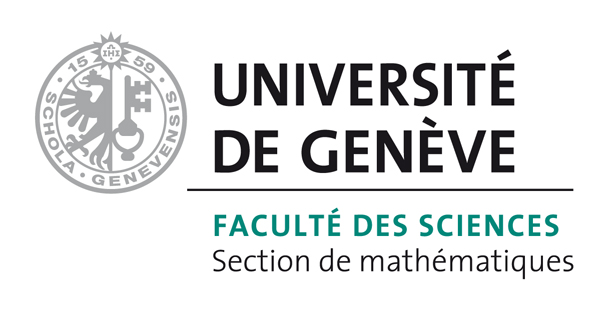# Bart Vandereycken## Distributed Principal Component Analysis with Limited Communication

by , , ,

#### Abstract:

We study efficient distributed algorithms for the fundamental problem of principal component analysis and leading eigenvector computation on the sphere, when the data are randomly distributed among a set of computational nodes. We propose a new quantized variant of Riemannian gradient descent to solve this problem, and prove that the algorithm converges with high probability under a set of necessary spherical-convexity properties. We give bounds on the number of bits transmitted by the algorithm under common initialization schemes, and investigate the dependency on the problem dimension in each case.

#### Reference:

F. Alimisis, P. Davies, B. Vandereycken, D. Alistarh, "Distributed Principal Component Analysis with Limited Communication", In 35th Conference on Neural Information Processing Systems (NeurIPS 2021), Sydney, Australia (to appear), 2021.

#### Bibtex Entry:

@inproceedings{Alimisis_DVA:2021,
title = {Distributed {{Principal Component Analysis}} with {{Limited Communication}}},
booktitle = {35th Conference on Neural Information Processing Systems (NeurIPS 2021), Sydney, Australia (to appear)},
author = {Alimisis, F. and Davies, P. and Vandereycken, B. and Alistarh, D.},
year = {2021},
abstract = {We study efficient distributed algorithms for the fundamental problem of principal component analysis and leading eigenvector computation on the sphere, when the data are randomly distributed among a set of computational nodes. We propose a new quantized variant of Riemannian gradient descent to solve this problem, and prove that the algorithm converges with high probability under a set of necessary spherical-convexity properties. We give bounds on the number of bits transmitted by the algorithm under common initialization schemes, and investigate the dependency on the problem dimension in each case.},
Pdf = {http://www.unige.ch/math/vandereycken/papers/published_Alimisis_DVA.pdf}
}